Nine words, nine Processing sketches

Following on from my nine images and one video, the next part of the brief was to use Processing to create a response to the same nine words. I’ve included the code for each, as per the brief, although WordPress unfortunately mangles Processing’s nice auto formatting. It also makes this post very long, but you can skip through all the code sections if you’re so inclined. Without further ado…

1: Serendipity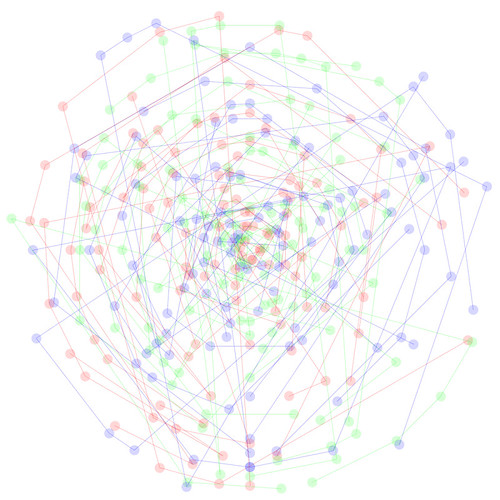I mentioned this in a previous post, but here it is again for good measure. It’s a visualisation of the numbers drawn over fifty draws of the National Lottery. Here’s the Processing code for it, although it won’t work without the data file.

```String[] lines; String[] numbers1; int[] numbers; float xpos; float ypos;```

``` void setup() { size(1000, 1000); lines = loadStrings("numbers.txt"); background(255); smooth(); } void draw() { background(255); for(int j=0; j<lines.length; j++) { //run through every line of data numbers1= splitTokens(lines[j]); //separate each line into its component strings numbers= int(numbers1); //convert string[] to int[] float distance= map(j, 0, lines.length, 20, width/2); beginShape(); for (int i=0; i<numbers.length; i++) { //run through each individual data point if(j%3 == 0) { fill(255, 0, 0, 40); } else if(j%3 ==1) { fill(0, 255, 0, 40); } else if(j%3 ==2) { fill(0, 0, 255, 40); } float angle= map(numbers[i], 1, 49, 0, TWO_PI); float x= (width/2)+sin(angle)*distance; float y= (height/2)+cos(angle)*distance; xpos= map(numbers[i], 1, 49, 50, width-50); noStroke(); ellipse(x, y, 20, 20); noFill(); if(j%3 == 0) { stroke(255, 0, 0, 70); } else if(j%3 ==1) { stroke(0, 255, 0, 70); } else if(j%3 ==2) { stroke(0, 0, 255, 70); } vertex(x, y); } endShape(); } } ```

```void keyPressed() { if(key=='s') { saveFrame("lotteryViz4.tif"); } }```

2: Sequential
This sketch divides the letters from a given word or phrase and rearranges them in 3d space, joined by bezier curves. This uses the PeasyCam library for camera control.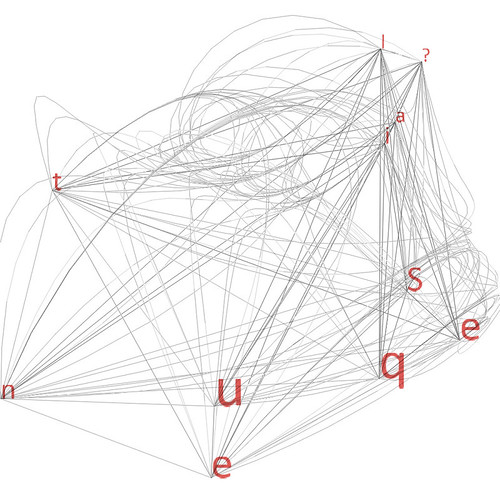``` import peasy.*;```

``` PeasyCam cam; PFont f; float[] ptA; float[] ptB; float[] ptC; float noiseSourceA= 0; float noiseSourceB= 0; float noiseSourceC= 0; float mod= 0.5; int iterations=1; String message= "Sequential?"; int maxIterations= message.length(); void setup() { size(1000, 1000, P3D); smooth(); cam = new PeasyCam(this, 600); f= loadFont("Calibri-72.vlw"); textFont(f, 25); ptA= new float; ptB= new float; ptC= new float; } void draw() { background(255); if(iterations<=maxIterations) { float a= calc(noiseSourceA); float b= calc(noiseSourceB); float c= calc(noiseSourceC); ptA= append(ptA, a); ptB= append(ptB, b); ptC= append(ptC, c); noiseSourceA+= random(mod); noiseSourceB+= random(mod); noiseSourceC+= random(mod); } fill(210, 17, 12, 180); for (int i=0; i<ptA.length; i++) { text(message.charAt(i%message.length()), ptA[i], ptB[i], ptC[i]); println(ptA.length); } noFill(); stroke(0, 50); beginShape(); for(int i=0; i<ptA.length; i++) { for (int j=0; j<ptA.length; j++) { vertex(ptA[i], ptB[i], ptC[i]); vertex(ptA[j], ptB[j], ptC[j]); bezierVertex(ptB[i], ptC[i], ptA[i], ptC[i], ptA[i], ptB[i], ptA[i], ptB[i], ptC[i]); bezierVertex(ptB[j], ptC[j], ptA[j], ptC[j], ptA[j], ptB[j], ptA[j], ptB[j], ptC[j]); } } endShape(); if (iterations<= maxIterations) { iterations++; } } float calc(float source) { float noiseResult= noise(source); float noiseMap= map(noiseResult, 0, 1, -width/2, width/2); return noiseMap; } void keyPressed() { if(key==' ') { iterations=1; ptA= new float; ptB= new float; ptC= new float; } ```

``` if(key=='s') { saveFrame(); } } ```
3: Loop
This uses a several looping sections of code to create a repeating pattern controlled by trigonometry. Changing the values of x and y in the code produces radically different designs. I spent a morning at the Glasgow School of Art this week, then came home and developed this which, completely coincidentally, bears a certain resemblance to Charles Rennie Mackintosh’s geometric roses. Art imitating life?``` float x; float y; void setup() { size(1000, 1000); background(255); smooth(); vars(); } void vars() { x= width/2; y=width/2; } void draw() { println(x); noLoop(); //background(255); //noFill(); stroke(25, 50); for(int a=0; a<700; a++) { for(int i=0; i<10; i++) { fill(i*25, 0, 50, 25); float xx=map(sin(x), -1, 1, 0, width); float yy= map(cos(y), -1, 1, 0, height); ```

``` ellipse(xx, yy, (tan(i)*x%60), (tan(i)*y%50)); } x+=9; y+=7; } // saveFrame(); }```
4: Crash
I’ve been trying to use less random or pseudo-random (e.g. Perlin noise) functions in my coding but this seemed like the right place to use some randomness. This takes an image (a screenshot from one of my earlier posts) and chops random pieces out and rearranges them over the screen, collage style. I like how there is still recognisable chunks of the interface present despite the mangling.``` PImage img;```

``` void setup() { size(1000, 1000); img= loadImage("VerdantScapeScreen.jpg"); noLoop(); } int x=0; int y=0; ```

```void draw() { background(0); for(int a=0; awidth) { x=0; y+=int(random(50)); } } //saveFrame("###"); }```
5: Ambiguity
This sketch mashes up two images from my lottery visualisations post by taking alternate pixels from each image and combining them. This process can bring some pretty mad results depending on what pictures you use as sources. I rather like this one as it combines two pictures with definite meanings into one with no discernible meaning!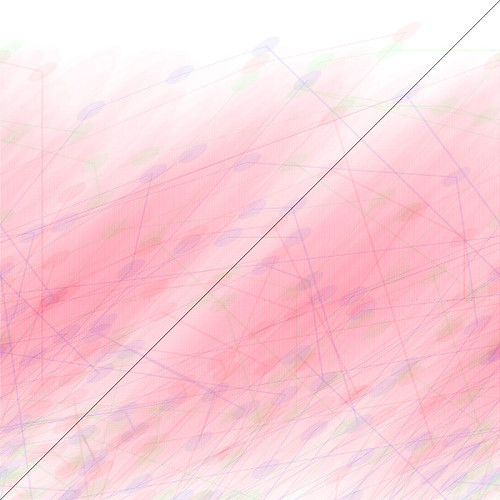```int x=0; int y=0; int a=0; int b=0;```

``` void setup() { size(1000, 1000, P2D); background(255); } void draw() { PImage img1= loadImage("lotteryViz2.jpg"); PImage img2= loadImage("lotteryviz4.jpg"); for(int i=0; iimg1.width) { x=0; y++; } if(a>width) { a=0; b++; } } } ```

```void keyPressed() { if (key=='s') { saveFrame("###.tif"); println("saved"); } }```
6: Condition
This uses the very cool Geomerative library which lets you break down shapes (including fonts and vector graphics) into points. This is actually a still from an animated version which seems to summarise the sketchy pencil feel you can achieve. If you run the code, you can switch the drawing style using the space bar.``` import geomerative.*;```

``` RFont f; RShape grp; RShape grp2; RPoint[] points; RPoint[] points2; boolean up= false; int polyLength= 0; boolean clear= false; void setup() { size(1000, 600, P2D); frameRate(20); background(255); RG.init(this); grp = RG.getText("Condition:", "GenBasB.ttf", 150, CENTER); grp2= RG.getText("sketchy", "GenBasB.ttf", 150, CENTER); smooth(); } void draw() { if(clear==true) { background(255); stroke(50, 150); } else { stroke(50, 10); } translate(width/2, height/2); RG.setPolygonizerLength(polyLength); points= grp.getPoints(); points2= grp2.getPoints(); for(int i=4; i<points.length-1; i++) { for(int j=0; j<5; j++) { line( points[i-j].x, points[i-j].y, points[i].x, points[i].y); } } translate(0, 200); for(int i=4; i<points2.length-1; i++) { for(int j=0; j<5; j++) { line( points2[i-j].x, points2[i-j].y, points2[i].x, points2[i].y); } } if(up==false) { polyLength--; } else { polyLength++; } if(polyLength255) { up=!up; background(255); } } ```

```void keyPressed() { if(key==' ') { clear=!clear; } if(key=='s') { saveFrame("###"); } }```
7: Diaphanous
This one is pretty similar to some of my previous Perlin noise experiments, but it uses bezier curves which give it a lovely organic feel. It uses the OpenGL renderer as its painfully slow otherwise.``` import processing.opengl.*;```

``` ArrayList beziers; color red1= color(237, 12, 12); color blue1= color(71, 2, 201); float con1xSource=random(100); float con1ySource=random(100); float con2xSource=random(100); float con2ySource=random(100); float point1xSource=random(100); float point1ySource=random(100); float point2xSource=random(100); float point2ySource=random(100); float transStroke= 30; float transFill= 4; float mod= 0.007; void setup() { size(1000, 1000, OPENGL); smooth(); background(255); beziers= new ArrayList(); } void draw() { background(255); for(int i= 1; i<beziers.size(); i++) { FadingBezier theBeziers= (FadingBezier) beziers.get(i); theBeziers.drawCurve(); theBeziers.update(); if(theBeziers.strokeTrans<0) { beziers.remove(i); } } println(beziers.size()); float con1x= calc(con1xSource); float con1y= calc(con1ySource); float con2x= calc(con2xSource); float con2y= calc(con2ySource); float point1x= calc(point1xSource); float point1y= calc(point1ySource); float point2x= calc(point2xSource); float point2y= calc(point2ySource); beziers.add(new FadingBezier(0, point2y, con1x, con1y, con2x, con2y, point1x, point1y, transStroke, transFill, red1, blue1)); beziers.add(new FadingBezier(width, point2x, con1x, con1y, con2x, con2y, point1x, point1y, transStroke, transFill, red1, blue1)); beziers.add(new FadingBezier(point2x, 0, con1x, con1y, con2x, con2y, point1x, point1y, transStroke, transFill, blue1, red1)); beziers.add(new FadingBezier(point2y, height, con1x, con1y, con2x, con2y, point1x, point1y, transStroke, transFill, blue1, red1)); con1xSource+=random(mod); con1ySource+=random(mod); con2xSource+=random(mod); con2ySource+=random(mod); point1xSource+=random(mod); point1ySource+=random(mod); point2xSource+=random(mod); point2ySource+=random(mod); } float calc(float source) { float noiseResult= noise(source); float noiseMap= map(noiseResult, 0, 1, 0, width); return noiseMap; } void mousePressed() { beziers= new ArrayList(0); } void keyPressed(){ if(key=='s'){ saveFrame("beziers###.tif"); } } class FadingBezier { float x1, y1, c1x, c1y, c2x, c2y, x2, y2, strokeTrans, fillTrans; color strokeCol, fillCol; FadingBezier(float X1, float Y1, float C1X, float C1Y, float C2X, float C2Y, float X2, float Y2, float STROKETRANS, float FILLTRANS, color STROKECOL, color FILLCOL) { x1= X1; y1= Y1; c1x= C1X; c1y= C1Y; c2x= C2X; c2y= C2Y; x2= X2; y2= Y2; strokeTrans= STROKETRANS; fillTrans= FILLTRANS; strokeCol= STROKECOL; fillCol= FILLCOL; } void drawCurve() { stroke (strokeCol, strokeTrans); fill(fillCol, fillTrans); bezier(x1, y1, c1x, c1y, c2x, c2y, x2, y2); } ```

``` void update() { strokeTrans-= 0.1; fillTrans-= 0.09; } } ```
8: Utopia
OK, time to push the boat out slightly. Number eight is a rendering of an IDICwhich is a symbol of the logical foundations of Vulcan philosophy from Star Trek. It also inspired the sub-title for this blog: Infinite Diversity in Infinite Combination. For me this is the ideal way of approaching life and art, especially when it comes to coding and generative art, although I should stress that I liked logic before I liked Star Trek! Geek out. I looked at this as an exercise in making sure this design scales to fit the window- as long as its square, it should display properly regardless of size.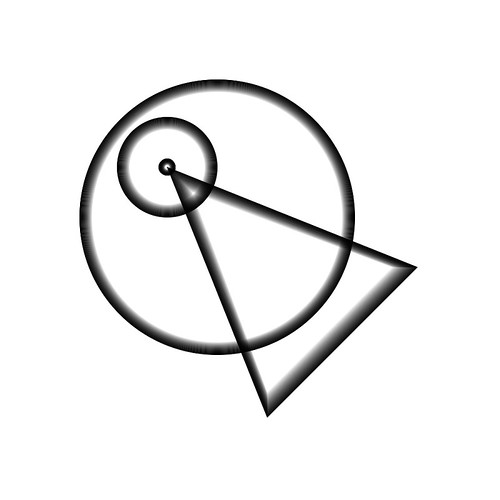``` void setup() { size(1000, 1000); smooth(); background(255); }```

``` void draw() { translate(-width/6, -width/6); background(255); noFill(); for(int i=0; i<50; i++) { stroke(0, 255-(i*5)); ellipse(width*0.6, width*0.6, (width*0.55)-i, (width*0.55)-i); ellipse(width/2, width/2, (width/5)-i, (width/5)-i); ellipse(width/2, width/2, (width/30)-i/2, (width/30)-i/2); triangle(width/2+i, width/2+i, width-i, width*0.7, width*0.7, width-i); } } ```

```void keyPressed() { if(key=='s') { saveFrame("###"); } }```
9: Ephemeral
Finally for now, another bit of sci-fi homage. Inspired by this fantastic scene from the end of Blade Runner, I wanted to try and capture the spirit of Rutger Hauer’s monologue. That scene is mainly defined by his phrasing, which is so difficult to capture in text, but I tried to capture other elements: the dove escaping as he dies, the idea of burning out rather than fading away. I had a much busier version which I actually uploaded (its on my Flickr if you want to look for it) but this one works better I think. The code is pretty lengthy/clumsy as I’ve not yet found a way to process the three arrays of points together. Again, if you want to run the code you’ll need to get the Geomerative library.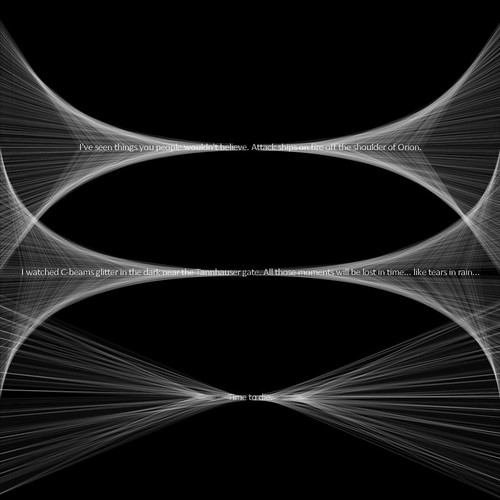``` import geomerative.*;```

``` RShape grp0; RShape grp1; RShape grp2; RPoint[] points0; RPoint[] points1; RPoint[] points2; String[] cbeams; PFont f; void setup() { size(1000, 1000); cbeams= loadStrings("cBeams.txt"); RG.init(this); f= loadFont("Calibri-48.vlw"); grp0= RG.getText(cbeams, "calibri.ttf", 15, CENTER); grp1= RG.getText(cbeams, "calibri.ttf", 15, CENTER); grp2= RG.getText(cbeams, "calibri.ttf", 15, CENTER); RG.setPolygonizerLength(2); points0= grp0.getPoints(); RG.setPolygonizerLength(3); points1= grp1.getPoints(); RG.setPolygonizerLength(1); points2= grp2.getPoints(); textFont(f, 20); textAlign(CENTER); background(0); smooth(); println(points2.length); strokeWeight(0.7); } void draw() { noLoop(); background(0); noStroke(); fill(255, 150); pushMatrix(); translate(width*0.5, height*0.3); grp0.draw(); for(int i=0; i<points0.length; i++) { stroke(255, 10); float offset=map(i, 0, points0.length, -250, 250); if(points0[i].x0) { line(points0[i].x, points0[i].y, width/2, points0[i].y+offset); line(points0[i].x, points0[i].y, width/2, points0[i].y-offset); } } translate(0, height*0.25); for(int i=0; i<points1.length; i++) { stroke(255, 10); float offset=map(i, 0, points1.length, 250, -250); if(points1[i].x0) { line(points1[i].x, points1[i].y, width/2, points1[i].y+offset); line(points1[i].x, points1[i].y, width/2, points1[i].y-offset); } } noStroke(); fill(255, 150); grp1.draw(); translate(0, height*0.25); for(int i=0; i<points2.length; i++) { stroke(255, 10); float offset=map(i, 0, points2.length, 200, -200); if(points2[i].x0) { line(points2[i].x, points2[i].y, width/2, points2[i].y+offset); line(points2[i].x, points2[i].y, width/2, points2[i].y-offset); line(points2[i].x+60, points2[i].y, width/2, points2[i].y+offset); line(points2[i].x+60, points2[i].y, width/2, points2[i].y-offset); } } noStroke(); fill(255, 150); grp2.draw(); popMatrix(); ```

``` //saveFrame("###"); }```
So, there we are. Please let me know what you think of these pieces, or if you have any questions or suggestions. Until next time, live long and prosper!